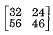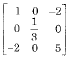# determinant of the matrix is

1.  -76

2.  -28

3.  +28

4.  +72

4

-28

Explanation :
No Explanation available for this question

# the product [P][Q]T of the following two matrices [P] and [Q] is [P]= ;[Q]=

1.

2.

3.

4.

4Explanation :
No Explanation available for this question

# eigen values of the matrix are

1.  3 and -5

2.  -3 and 5

3.  -3 and -5

4.  3 and 5

4

3 and -5

Explanation :
No Explanation available for this question

# A set of linear equations is represented by the matrix equation Ax=b. the necessary condition for the existence of a solution for this system is

1.  A must be invertible

2.  B must be linearly dependent of the columns of A

3.  B must be linearly independent of the columns of A

4.  None of these

4

B must be linearly dependent of the columns of A

Explanation :
No Explanation available for this question

# the vector is an eigen vector of A= One of the eigen values of A is

1.  1

2.  2

3.  5

4.  -1

4

5

Explanation :
No Explanation available for this question

# given;A= Some of the igen values of the matrix A is

1.  10

2.  -10

3.  24

4.  22

4

10

Explanation :
No Explanation available for this question

# A=.The inverse of A is

1.

2.

3.

4.

4Explanation :
No Explanation available for this question

# determinant of the matrix is

1.  100

2.  200

3.  1

4.  300

4

100

Explanation :
No Explanation available for this question

# the matrix obtained from a given matrix by changing rows into columns is called

1.  Involutory matrix

2.  Orthogonal matrix

3.  Symmetric matrix

4.  None of these

4

Symmetric matrix

Explanation :
No Explanation available for this question

# if AB=BA, then AB is a symmetric matrix, if A and B are

1.  Symmetric matrix

2.  Skew symmetric matrix

3.  Orthogonal matrix

4.  None of these

4